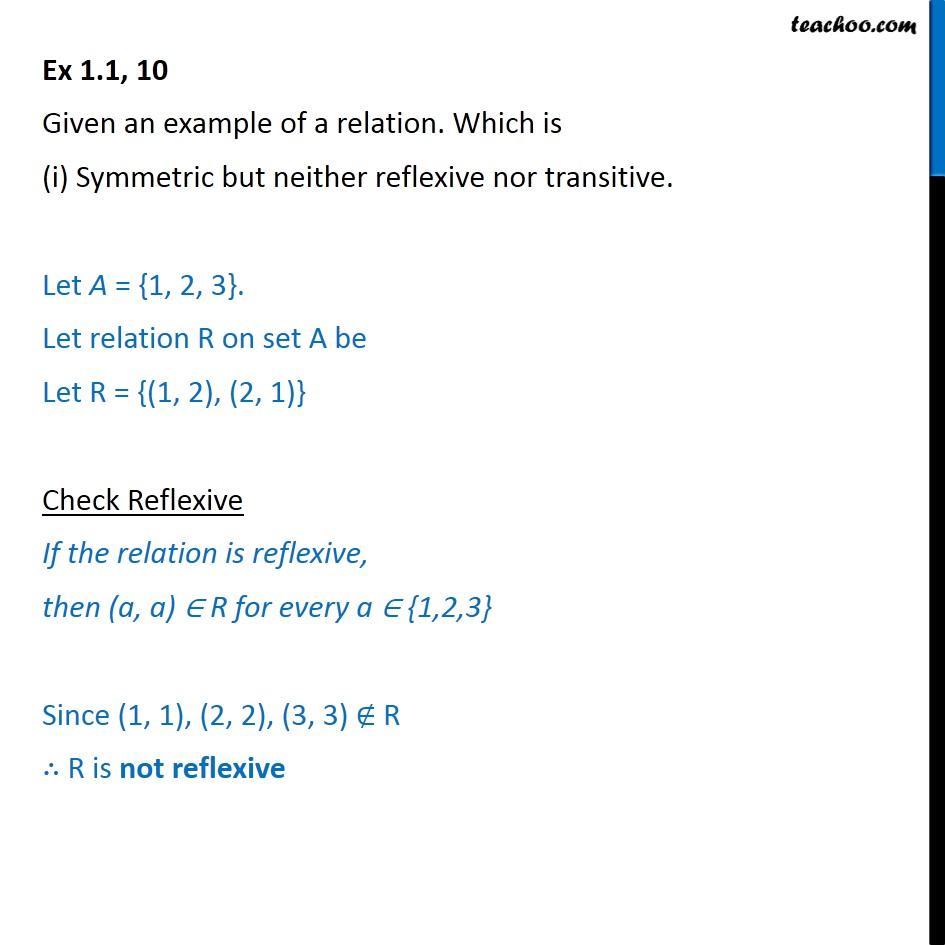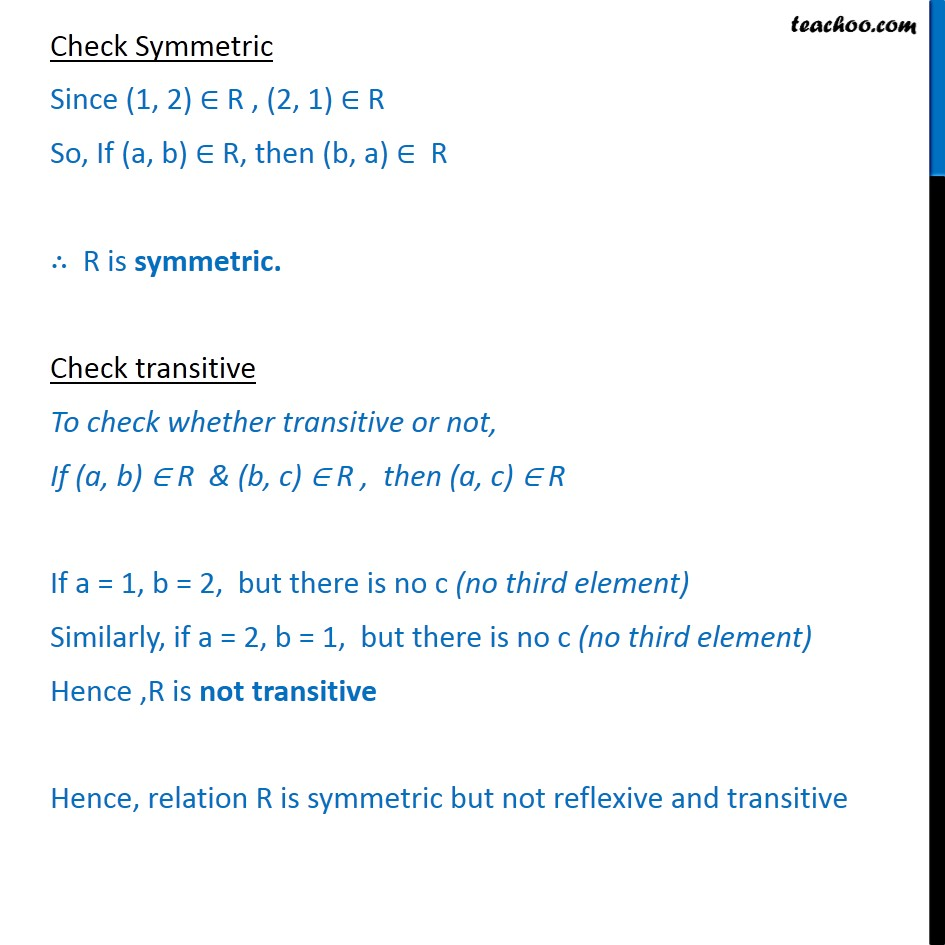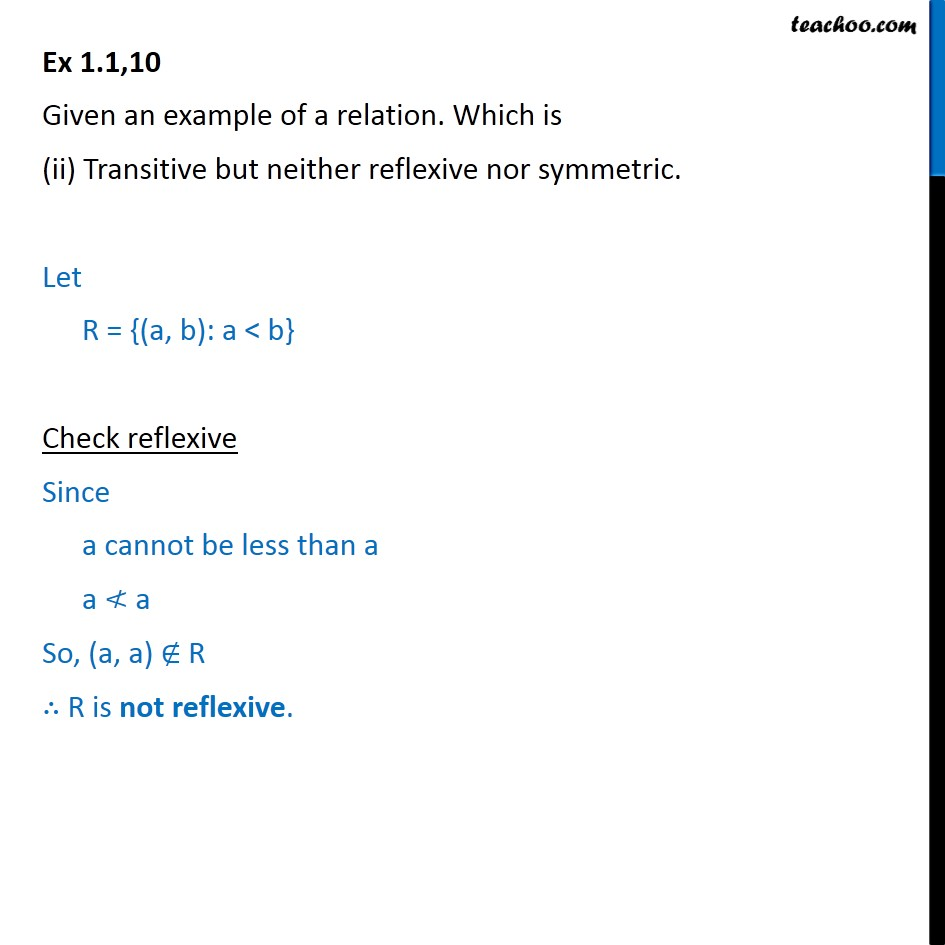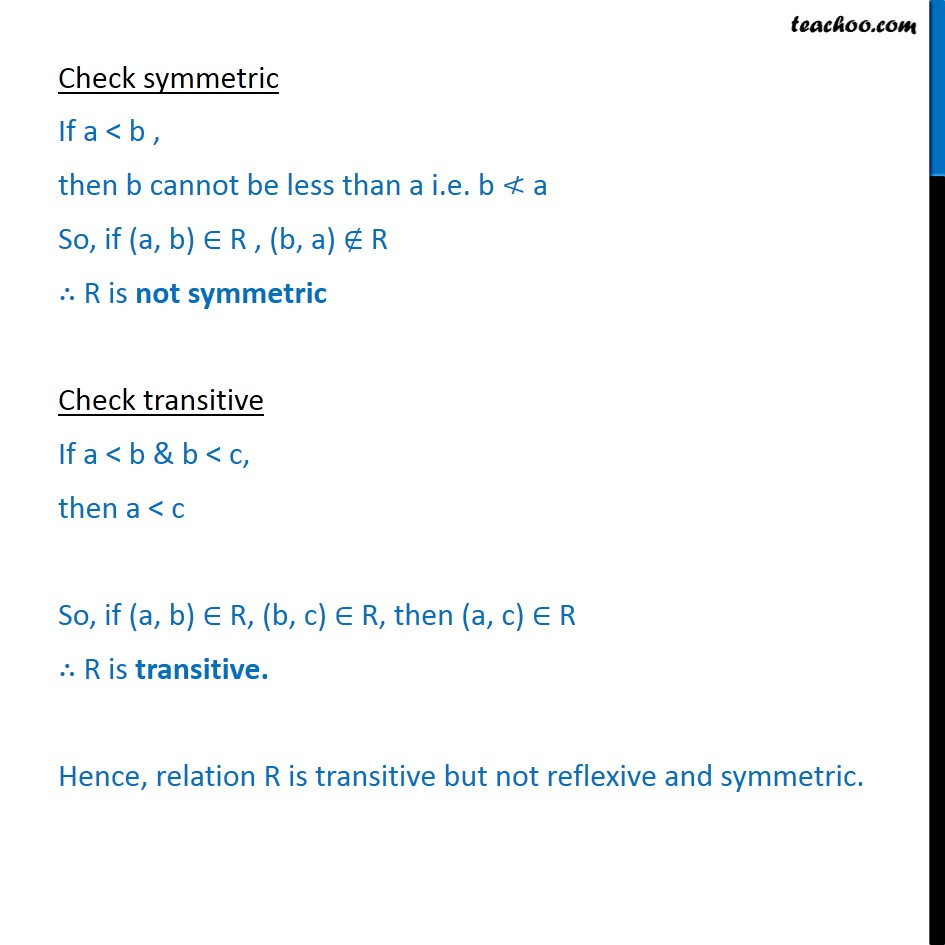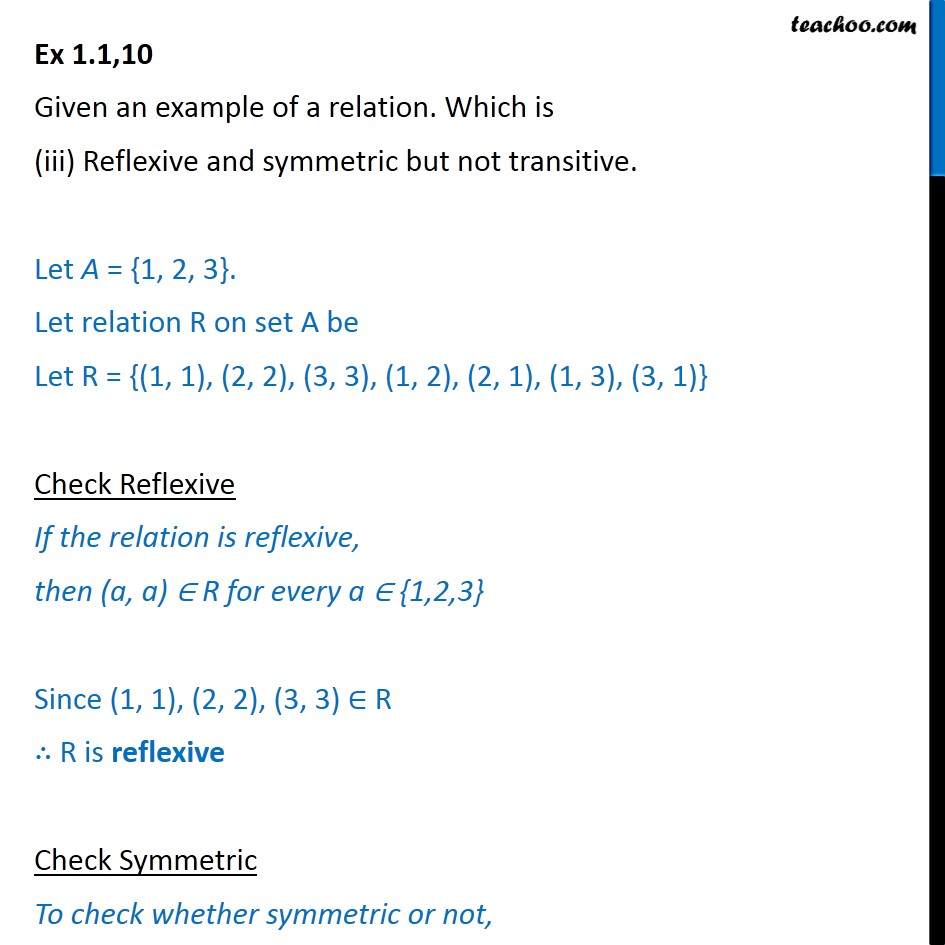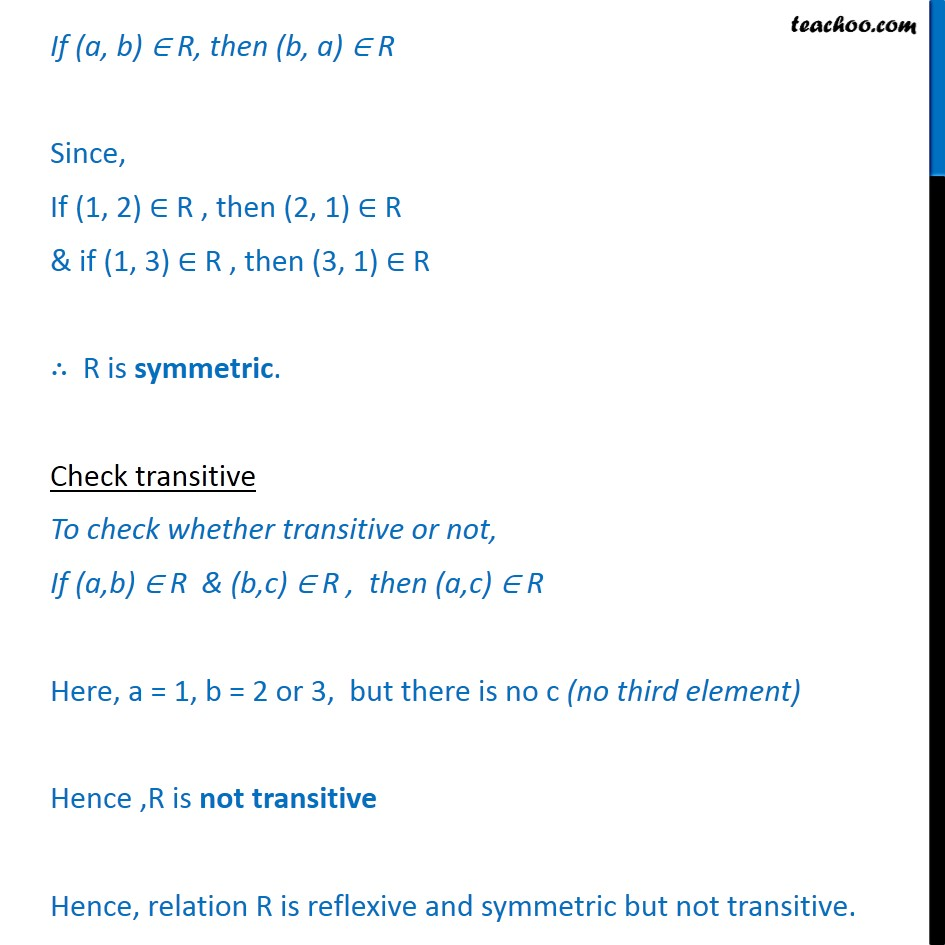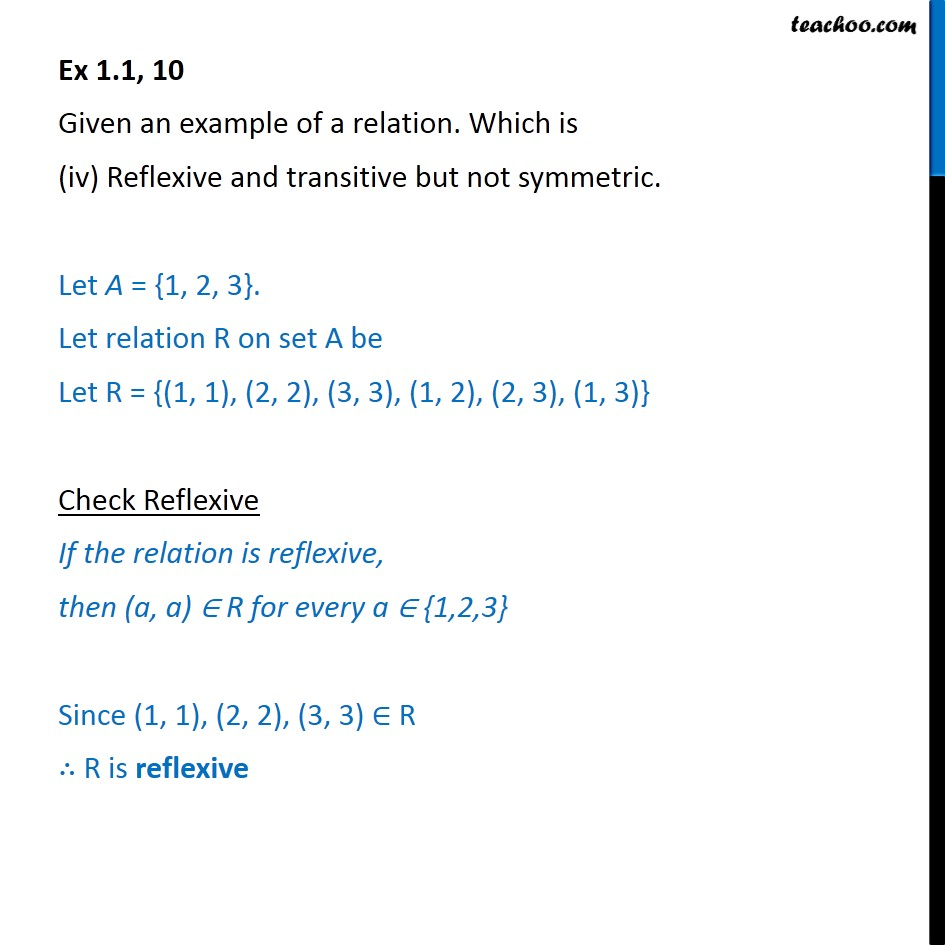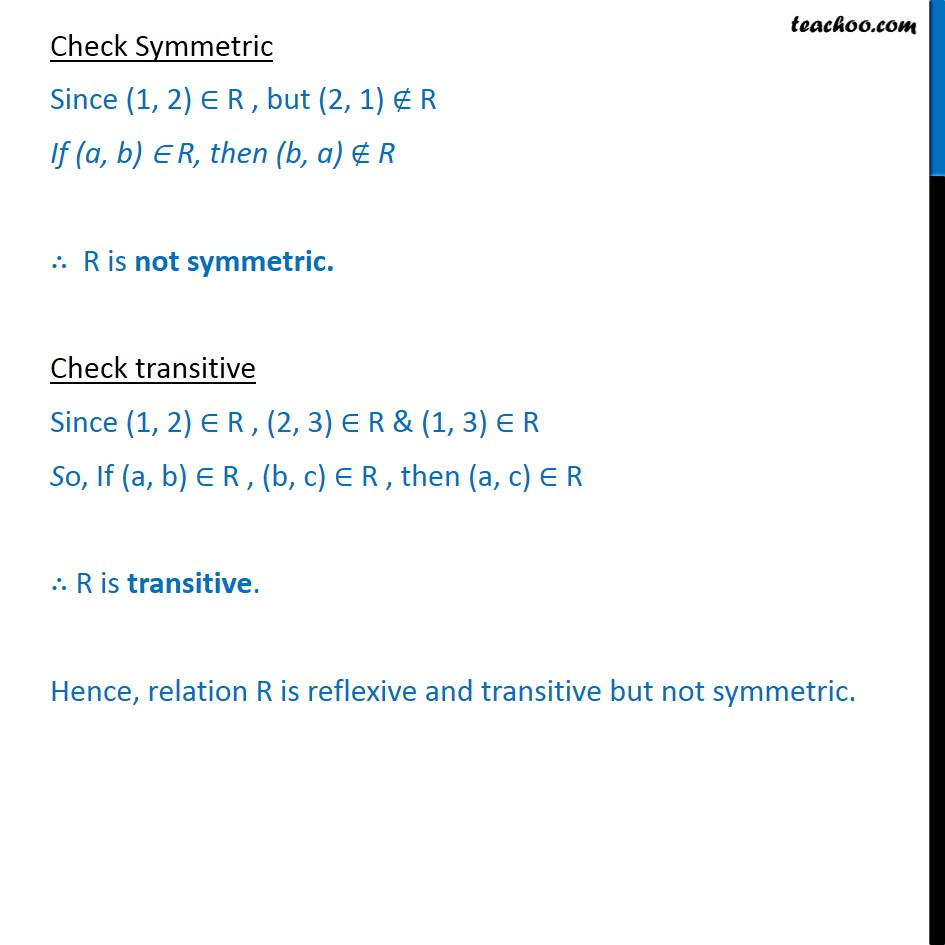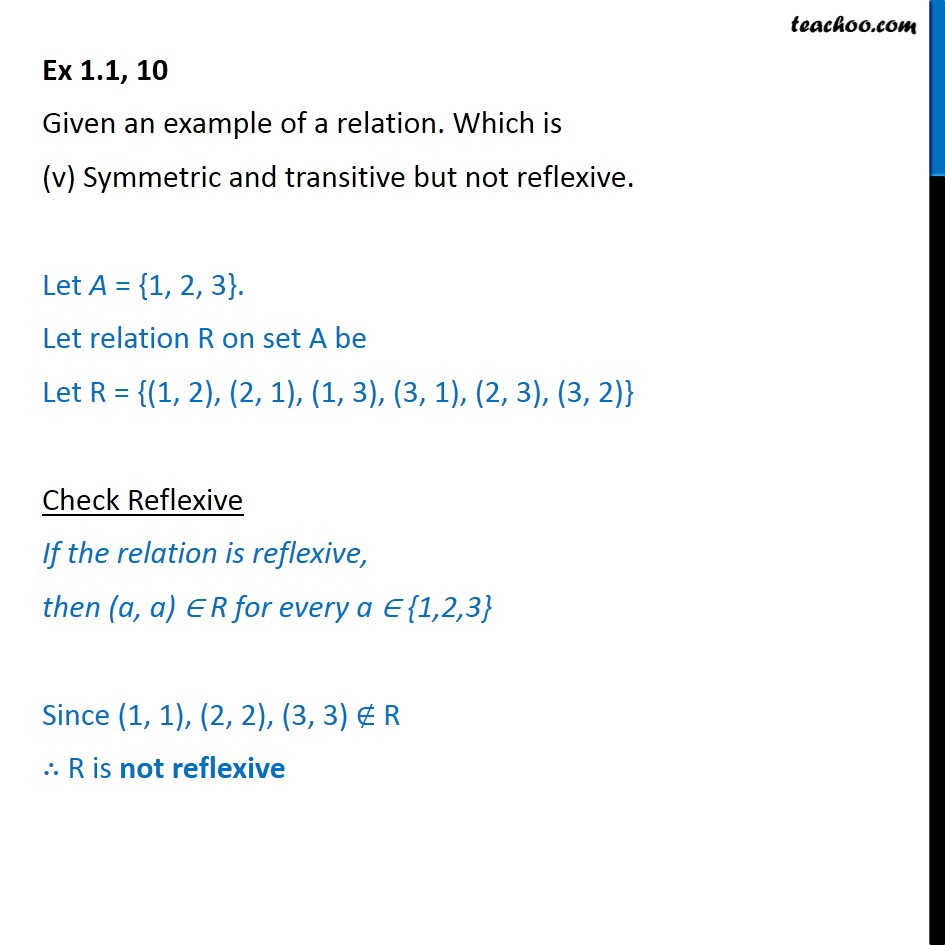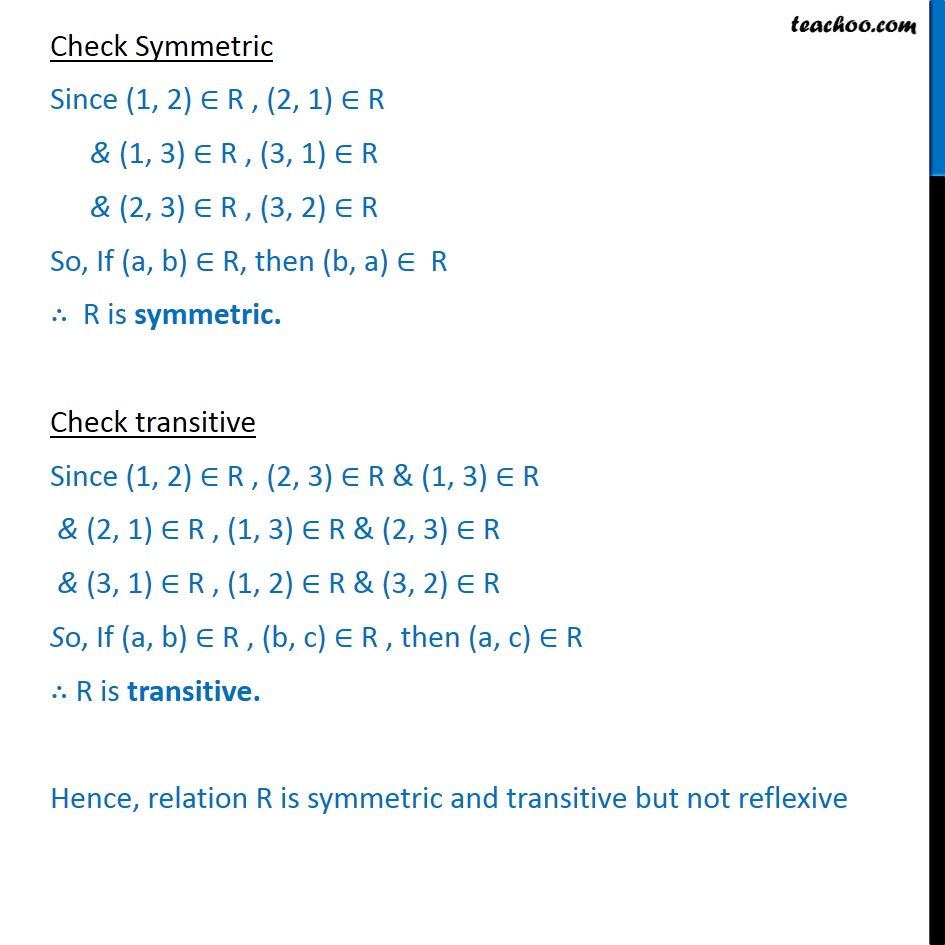Subscribe to our Youtube Channel - https://you.tube/teachoo

1. Chapter 1 Class 12 Relation and Functions
2. Serial order wise
3. Ex 1.1

Transcript

Ex 1.1, 10 Given an example of a relation. Which is (i) Symmetric but neither reflexive nor transitive. Let A = {1, 2, 3}. Let relation R on set A be Let R = {(1, 2), (2, 1)} Check Reflexive If the relation is reflexive, then (a, a) R for every a {1,2,3} Since (1, 1), (2, 2), (3, 3) R R is not reflexive Check Symmetric Since (1, 2) R , (2, 1) R So, If (a, b) R, then (b, a) R R is symmetric. Check transitive To check whether transitive or not, If (a, b) R & (b, c) R , then (a, c) R If a = 1, b = 2, but there is no c (no third element) Similarly, if a = 2, b = 1, but there is no c (no third element) Hence ,R is not transitive Hence, relation R is symmetric but not reflexive and transitive Ex 1.1,10 Given an example of a relation. Which is (ii) Transitive but neither reflexive nor symmetric. Let R = {(a, b): a < b} Check reflexive Since a cannot be less than a a a So, (a, a) R R is not reflexive. Check symmetric If a < b , then b cannot be less than a i.e. b a So, if (a, b) R , (b, a) R R is not symmetric Check transitive If a < b & b < c, then a < c So, if (a, b) R, (b, c) R, then (a, c) R R is transitive. Hence, relation R is transitive but not reflexive and symmetric. Ex 1.1,10 Given an example of a relation. Which is (iii) Reflexive and symmetric but not transitive. Let A = {1, 2, 3}. Let relation R on set A be Let R = {(1, 1), (2, 2), (3, 3), (1, 2), (2, 1), (1, 3), (3, 1)} Check Reflexive If the relation is reflexive, then (a, a) R for every a {1,2,3} Since (1, 1), (2, 2), (3, 3) R R is reflexive Check Symmetric To check whether symmetric or not, If (a, b) R, then (b, a) R Since, If (1, 2) R , then (2, 1) R & if (1, 3) R , then (3, 1) R R is symmetric. Check transitive To check whether transitive or not, If (a,b) R & (b,c) R , then (a,c) R Here, a = 1, b = 2 or 3, but there is no c (no third element) Hence ,R is not transitive Hence, relation R is reflexive and symmetric but not transitive. Ex 1.1, 10 Given an example of a relation. Which is (iv) Reflexive and transitive but not symmetric. Let A = {1, 2, 3}. Let relation R on set A be Let R = {(1, 1), (2, 2), (3, 3), (1, 2), (2, 3), (1, 3)} Check Reflexive If the relation is reflexive, then (a, a) R for every a {1,2,3} Since (1, 1), (2, 2), (3, 3) R R is reflexive Check Symmetric Since (1, 2) R , but (2, 1) R If (a, b) R, then (b, a) R R is not symmetric. Check transitive Since (1, 2) R , (2, 3) R & (1, 3) R So, If (a, b) R , (b, c) R , then (a, c) R R is transitive. Hence, relation R is reflexive and transitive but not symmetric. Ex 1.1, 10 Given an example of a relation. Which is (v) Symmetric and transitive but not reflexive. Let A = {1, 2, 3}. Let relation R on set A be Let R = {(1, 2), (2, 1), (1, 3), (3, 1), (2, 3), (3, 2)} Check Reflexive If the relation is reflexive, then (a, a) R for every a {1,2,3} Since (1, 1), (2, 2), (3, 3) R R is not reflexive Check Symmetric Since (1, 2) R , (2, 1) R & (1, 3) R , (3, 1) R & (2, 3) R , (3, 2) R So, If (a, b) R, then (b, a) R R is symmetric. Check transitive Since (1, 2) R , (2, 3) R & (1, 3) R & (2, 1) R , (1, 3) R & (2, 3) R & (3, 1) R , (1, 2) R & (3, 2) R So, If (a, b) R , (b, c) R , then (a, c) R R is transitive. Hence, relation R is symmetric and transitive but not reflexive

Ex 1.1